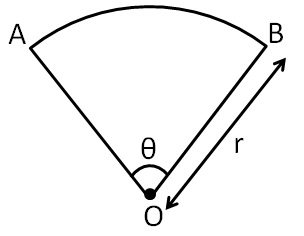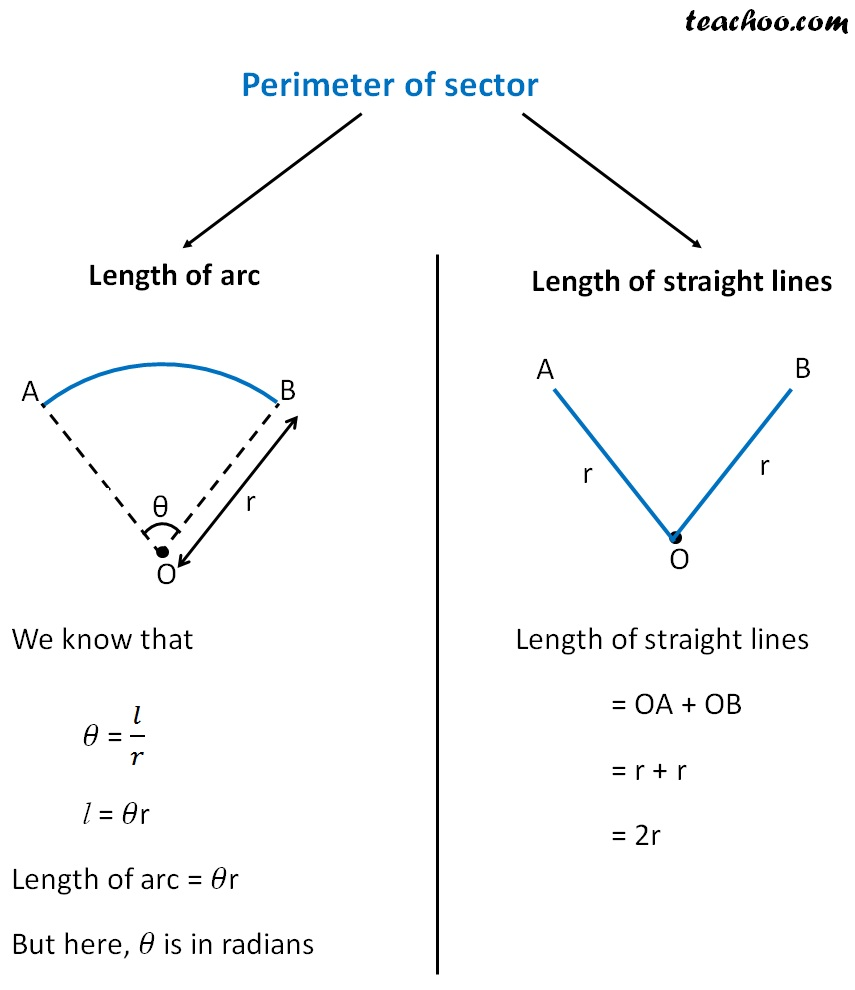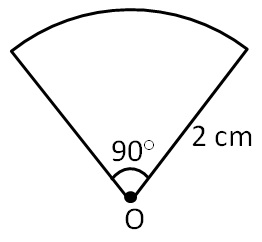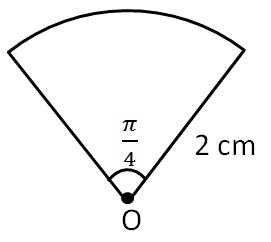Perimeter of a combination of shapes

Chapter 11 Class 7 Perimeter and Area
Concept wise

A sector is a part of the circlePerimeter of sector will be the distance around itThus,

Perimeter of sector = 𝜃r + 2r

= r(𝜃 + 2)

If angle is in degrees,

𝜃 = Angle × π/(180°)

Let us take some examples:

#### Find perimeter of sector whose radius is 2 cm and angle is of 90°First,

We need to convert angle in radians

𝜃 = Angle in degree × π/(180°)

𝜃 = 90° × π/(180° )

𝜃 = π/4

= 2 cm

Now,

Perimeter of sector  = r (𝜃 + 2)

= 2 (π/4+2)

= 2 ((π + 2 × 4)/4)

= 2 ((π + 8)/4)

= (π + 8)/4 cm

#### Find perimeter of sector whose radius is 2 cm and angle is π/4 radians= 2 cm

= π/4

Now,

Perimeter of sector = r (𝜃 + 2)

= 2 (π/4+2)

= 2 ((π + 2 × 4)/4)= 2 ((π + 8)/4) = (π + 8)/4 cm

Get live Maths 1-on-1 Classs - Class 6 to 12